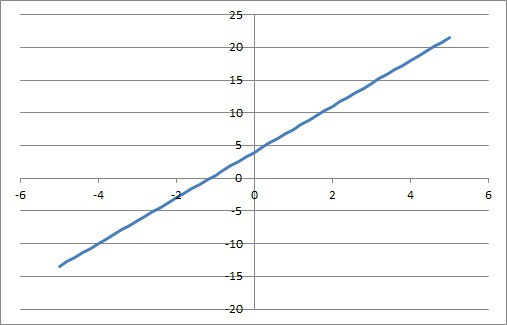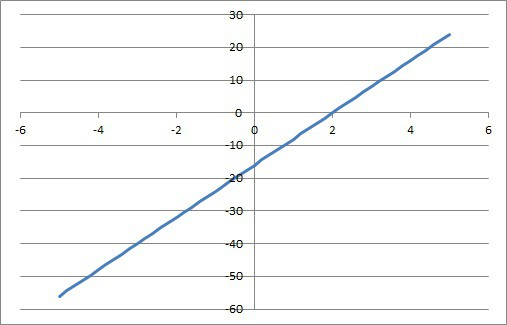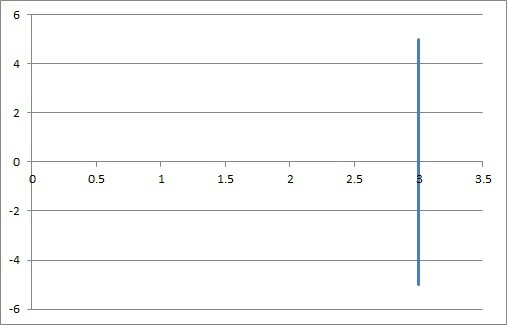# Difference Between Positive & Negative Slope (3 Key Ideas)

Slope is an important concept when it comes to working with lines and their graphs.  Knowing what the sign of the slope means will make it much easier to understand linear functions.

So, what is the difference between positive and negative slope?  A positive slope means y increases as x increases (visually, the line moves up as you go from left to right).  A negative slope means y decreases as x increases (visually, the line moves down as you go from left to right).  A zero slope means that y is constant and does not change as x changes.

Of course, a line can also have an undefined (or infinite) slope, and this happens in a certain specific case.

In this article, we’ll talk about the difference between positive and negative slope, along with zero and undefined slope.  We’ll also look at some examples to make the concept clear.

Let’s get started.

Having math trouble?

Looking for a tutor?

## Difference Between Positive & Negative Slope

The difference between positive and negative slope is what happens to y as x changes:

• Positive Slope: y increases as x increases.  (Alternatively, y decreases as x decreases.)  Visually, this means the line moves up as we go from left to right on the graph.
• Negative Slope: y decreases as x increases.  (Alternatively, y increases as x decreases.)  Visually, this means the line moves down as we go from left to right on the graph.
• Zero Slope: y stays the same as x increases.  (Alternatively, y stays the same as x decreases.)  Visually, this means the line is horizontal (a constant function): it does not move up or down as we go from left to right on the graph.
• Undefined (Infinite) Slope: y takes on any value and x takes on only one value.  Visually, this means the line is vertical.

The table below summarizes the information on slope:

Remember that we can also define slope algebraically as:

• Slope = rise/run, or
• Slope = (y2 – y1)/(x2 – x1)

This means that slope is a fraction whose numerator is the change in y and whose denominator is the change in x.  These are the cases for slope and what they mean for rise and run:

• When slope is positive, rise and run have the same sign (both are positive or both are negative).
• When slope is negative, rise and run have opposite signs (one is positive, the other is negative).
• When slope is zero, rise is zero and run is any nonzero value.
• When slope is undefined (infinite), run is zero and rise is any nonzero value.

The table below summarizes the signs of the slope depending on the signs of the rise and run.

Now let’s take a look at some examples of each type of slope.

### Positive Slope Examples

A line with a positive slope has a y-intercept (where x = 0) and an x-intercept (where y = 0), both of which could be any real number.  Let’s start with one that has a zero y-intercept.

#### Example 1: Positive Slope, Zero y-intercept

The line y = 2x has a positive slope (m = 2 is positive) and a zero y-intercept (b = 0).

This means that the line passes through the origin (0, 0).  In fact, the origin is both the y-intercept and the x-intercept for this line.

The slope of 2 can be rewritten as rise/run = 2/1.  This tells us that y increases by 2 every time x increases by 1.

The graph of the line y = 2x is shown below.

#### Example 2: Positive Slope, Positive y-intercept

The line y = 3.5x + 4 has a positive slope (m = 3.5 is positive) and a positive y-intercept (b = 4).

This means that the line passes through the point (0, 4), which is the y-intercept.

To find the x-intercept, we set y equal to zero and solve:

• y = 3.5x + 4  [line equation]
• 0 = 3.5x + 4  [set y to 0 to find x-intercept]
• -3.5x = 4
• x = -8/7

So, the x-intercept is the point (-8/7, 0).

The slope of 3.5 can be rewritten as rise/run = 3.5/1 = 7/2.  This tells us that y increases by 7 every time x increases by 2 (converting to whole numbers makes it easier to find another point on the line when graphing).

The graph of the line y = 3.5x + 4 is shown below.The graph of the line y = 3.5x + 4 with positive slope and positive y-intercept.

#### Example 3: Positive Slope, Negative y-intercept

The line y = 8x – 16 has a positive slope (m = 8 is positive) and a negative y-intercept (b = -16).

This means that the line passes through the point (0, -16), which is the y-intercept.

To find the x-intercept, we set y equal to zero and solve:

• y = 8x – 16  [line equation]
• 0 = 8x – 16  [set y to 0 to find x-intercept]
• -8x = -16
• x = 2

So, the x-intercept is the point (2, 0).

The slope of 8 can be rewritten as rise/run = 8/1.  This tells us that y increases by 8 every time x increases by 1.

The graph of the line y = 8x – 16 is shown below.The graph of the line y = 8x – 16 with positive slope and negative y-intercept.

Having math trouble?

Looking for a tutor?

### Negative Slope Examples

A line with a negative slope has a y-intercept and an x-intercept, both of which could be any real number.  Let’s start with one that has a zero y-intercept.

#### Example 1: Negative Slope, Zero y-intercept

The line y = -5x has a negative slope (m = -5 is negative) and a zero y-intercept (b = 0).

This means that the line passes through the origin (0, 0).  In fact, the origin is both the y-intercept and the x-intercept for this line.

The slope of -5 can be rewritten as rise/run = -5/1.  This tells us that y decreases by 5 every time x increases by 1.

The graph of the line y = -5xi s shown below.

#### Example 2: Negative Slope, Positive y-intercept

The line y = -2.75x + 3 has a negative slope (m = -2.75 is negative) and a positive y-intercept (b = 3).

This means that the line passes through the point (0, 3), which is the y-intercept.

To find the x-intercept, we set y equal to zero and solve:

• y = -2.75x + 3  [line equation]
• 0 = -2.75x + 3  [set y to 0 to find x-intercept]
• 2.75x = 3
• x = 12/11

So, the x-intercept is the point (12/11, 0).

The slope of -2.75 can be rewritten as rise/run = -2.75/1 = -11/4.  This tells us that y decreases by 11 every time x increases by 4 (converting to whole numbers makes it easier to find another point on the line when graphing).

The graph of the line y = -2.75x + 3 is shown below.

#### Example 3: Negative Slope, Negative y-intercept

The line y = -1.6x – 10 has a negative slope (m = -1.6 is negative) and a positive y-intercept (b = -10).

This means that the line passes through the point (0, -10), which is the y-intercept.

To find the x-intercept, we set y equal to zero and solve:

• y = -1.6x – 10  [line equation]
• 0 = -1.6x – 10  [set y to 0 to find x-intercept]
• 1.6x = -10
• x = -25/4

So, the x-intercept is the point (-25/4, 0).

The slope of -1.6 can be rewritten as rise/run = -1.6/10 = -4/25.  This tells us that y decreases by 4 every time x increases by 25 (converting to whole numbers makes it easier to find another point on the line when graphing).

The graph of the line y = -1.6x – 10 is shown below.

## What Is The Slope Of A Horizontal Line? (Zero Slope)

A horizontal line has a slope of zero (neither positive nor negative).  The y-intercept can be zero or nonzero, and the equation has the form

• y = b

where b is the y-intercept of the line, corresponding to the point (0, b).  Unless b = 0, a horizontal line will have no x-intercept.

If b = 0, the horizontal line will intersect the x-axis at every point.

### Example: Horizontal Line

For example, take the horizontal line y = 5.  It will never intersect the x axis, so it has no x-intercept.

The y-intercept is the point (0, 5).

The graph of the line y = 5 is shown below.

## What Is The Slope Of A Vertical Line? (Undefined Or Infinite Slope)

A vertical has an undefined or infinite slope.  It will have the equation x = a.

The x-intercept of vertical line will be the point (a, 0).  A vertical line will have no y-intercept unless a = 0, in which case the line intersects the y axis at every point.

### Example: Vertical Line

For example, take the vertical line x = 3.  It will never intersect the y axis, so it has no y-intercept.

The x-intercept is the point (3, 0).

The graph of the line x = 3 is shown below.The graph of the vertical line x = 3 with undefined (infinite) slope and no y-intercept.

## Conclusion

Now you know about the difference between positive and negative slope.  You also know when a zero or undefined slope occurs and what it means.